• 自己实现kmeans聚类算法matlab语言，带有界面，容易理解
运行界面（可以支持任意维数据）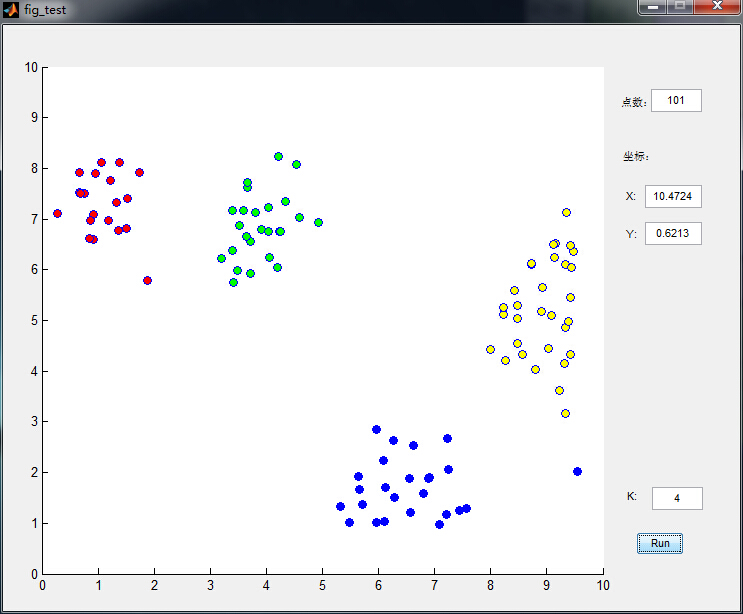单介绍下kmeans算法流程：

假设要把样本集分为c个类别，算法描述如下：

（1）适当选择c个类的初始中心；

（2）在第k次迭代中，对任意一个样本，求其到c各中心的距离，将该样本归到距离最短的中心所在的类；

（3）利用均值等方法更新该类的中心值；

（4）对于所有的c个聚类中心，如果利用（2）（3）的迭代法更新后，值保持不变，则迭代结束，否则继续迭代。

该算法的最大优势在于简洁和快速。算法的关键在于初始中心的选择和距离公式。

matlab实现：

function [ class count]=k_means(data,k);

%clear
%load testdata.mat
%k=2;
sum=size(data,1);
for i=1:k
centroid(i,:)=data(floor(sum/k)*(i-1)+1,:);
end
tic
ck=0;
while 1
temp=zeros(1,2);;
count=zeros(1,k);
ck=ck+1
for i=1:sum
for j=1:k
dist(j)=norm(data(i,:)-centroid(j,:));
end
[a min_dist]=min(dist);
count(min_dist)=count(min_dist)+1;
class(min_dist,count(min_dist))=i;
end

%重新计算类中心
for i=1:k
for j=1:count(i)
temp=temp+data(class(i,j),:);
end
temp_centroid(i,:)=temp/(count(i));
temp(1,:)=0;
% temp_centroid(i,:)=re_calculate(class(i,:),count(i),tdata);
end
%计算新的类中心和原类中心距离centr_dist;
for i=1:k
centr_dist(i)=norm(temp_centroid(i,:)-centroid(i,:));
end
if max(centr_dist)<=0
break;
else
for i=1:k
centroid(i,:)=temp_centroid(i,:);
%重新进行前俩不
end
end
end
toc


数据点是鼠标插进去的，通过界面可以很清晰的看到分类过程，功能截图如下：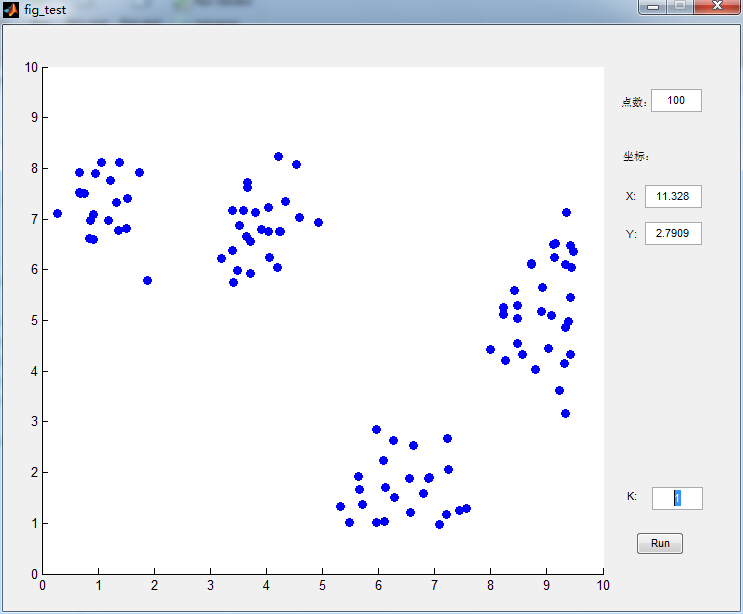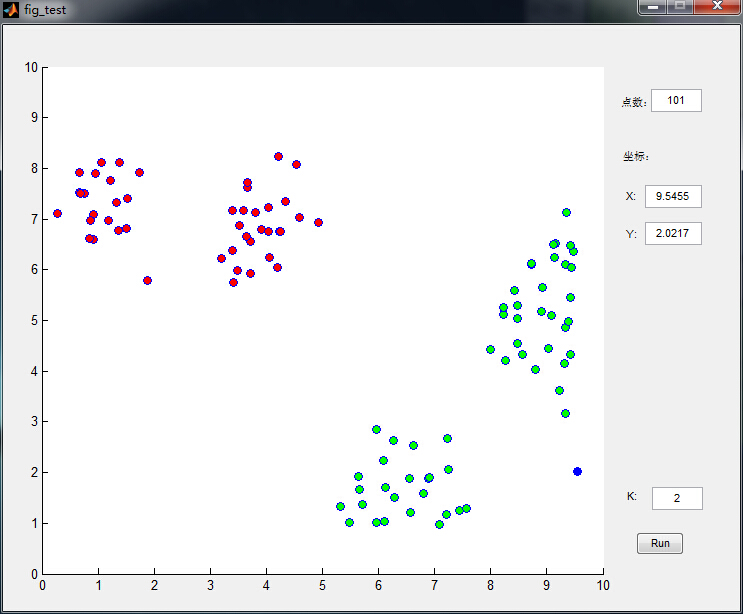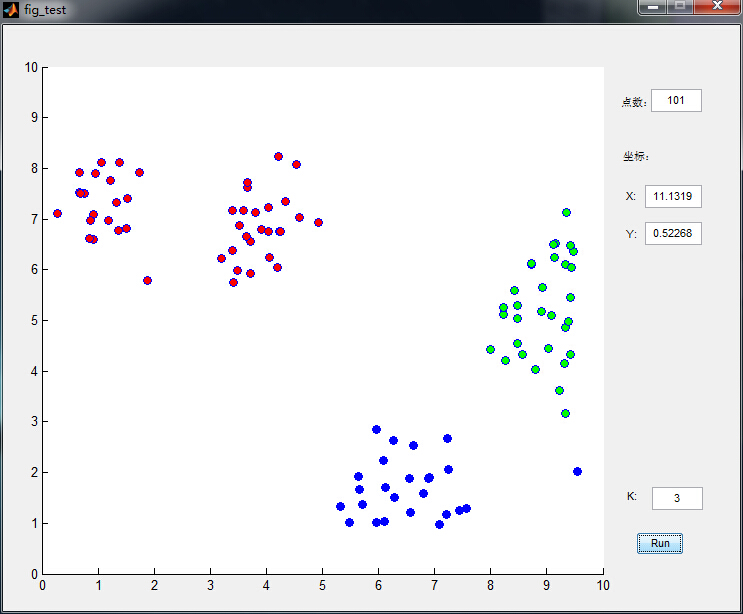源码连接：kmeans聚类算法源码


展开全文界面 数据
• ## Kmeans聚类算法详解

万次阅读 多人点赞 2018-05-16 18:41:40
摘要：本文通过图文详细介绍Kmeans聚类算法的原理和程序实现，以及如何选取类簇中心点。本文首先介绍利用该算法的原理及理解，详细介绍基于MATLAB设计一个自定义的Kmeans函数过程，然后利用该函数对UCI的数据集进行...
摘要：本文详细介绍Kmeans聚类算法的原理和程序实现。首先介绍利用该算法的原理及理解，详细介绍基于MATLAB设计一个自定义的Kmeans函数过程，然后利用该函数对UCI的数据集进行聚类以测试聚类结果。后续章节将介绍的主要部分有：

Kmeans算法的原理及理解
编程实现
聚类结果评价
类簇中心点的选取

点击下载：本文Kmeans算法M函数及测试完整文件1. 前言

作为无监督聚类算法中的代表——K均值聚类（Kmeans）算法，该算法的主要作用是将相似的样本自动归到一个类别中。所谓的监督算法，就是输入样本没有对应的输出或标签。聚类（clustering）试图将数据集中的样本划分为若干个通常是不相交的子集，每个子集称为一个“簇（cluster）”，聚类既能作为一个单独过程，用于找寻数据内在的分布结构，也可作为分类等其他学习任务的前去过程。——《Machine Learning》

聚类算法也许是机器学习中“新算法”出现最多、最快的领域，一个重要的原因是聚类不存在客观标准，给定数据集总能从某个角度找到以往算法未覆盖的某种标准从而设计出新算法。Kmeans算法十分简单易懂而且非常有效，但是合理的确定K值和K个初始类簇中心点对于聚类效果的好坏有很大的影响。众多的论文基于此都提出了各自行之有效的解决方案，新的改进算法仍然不断被提出，此类文章大家可以在Web Of Science中搜索。
尽管Kmeans算法在MATLAB、Python等语言的工具箱函数中都有自带的函数可供调用，但作为机器学习的研究者新来说要设计出新的算法，有时就得“定制”自己的Kmeans函数了。自己动手编写无疑也更加能理解算法的具体过程，接下来就让我们进入正题吧

2. Kmeans算法的原理与理解
Kmeans算法是最常用的聚类算法，主要思想是:在给定K值和K个初始类簇中心点的情况下，把每个点(亦即数据记录)分到离其最近的类簇中心点所代表的类簇中，所有点分配完毕之后，根据一个类簇内的所有点重新计算该类簇的中心点(取平均值)，然后再迭代的进行分配点和更新类簇中心点的步骤，直至类簇中心点的变化很小，或者达到指定的迭代次数。
2.1 基本原理
假定给定数据样本X，包含了n个对象$X=\left \{ X_{1} ,X_{2},X_{3},...,X_{n}\right \}$,其中每个对象都具有m个维度的属性。Kmeans算法的目标是将n个对象依据对象间的相似性聚集到指定的k个类簇中，每个对象属于且仅属于一个其到类簇中心距离最小的类簇中。对于Kmeans，首先需要初始化k个聚类中心$\left \{ C_{1} ,C_{2},C_{3},...,C_{k}\right \},1,然后通过计算每一个对象到每一个聚类中心的欧式距离，如下式所示$dis(X_{i},C_{j})=\sqrt{\sum_{t=1}^{m}(X_{it}-C_{jt})^{2}}$
上式中，$X_{i}$表示第i个对象$1\leq i\leq n$,$C_{j}$表示第j个聚类中心的$1\leq j\leq k$，$X_{it}$表示第i个对象的第t个属性，$1\leq t\leq m$，$C_{jt}$表示第j个聚类中心的第t个属性。
依次比较每一个对象到每一个聚类中心的距离，将对象分配到距离最近的聚类中心的类簇中，得到k个类簇$\left \{ S_{1},S_{2},S_{3},...,S_{k} \right \}$

Kmeans算法用中心定义了类簇的原型，类簇中心就是类簇内所有对象在各个维度的均值，其计算公式如下$C_{t}=\frac{\sum_{X_{i}\in S_{l}}X_{i}}{\left | S_{l} \right |}$
式中，$C_{l}$表示第l个聚类的中心，$1\leq l\leq k$，$\left | S_{l} \right |$表示第l个类簇中对象的个数，$X_{i}$表示第l个类簇中第i个对象，$1\leq i\leq\left | S_{l} \right |$。
2.2 算法流程

输入：样本集$D=\left \{ x_{1},x_{2},x_{3},...,x_{m} \right \}$；聚类簇数k.
过程：
1：从D中随机选择k个样本作为初始均值向量$\left \{ \mu _{1},\mu _{2},\mu _{3},...,\mu _{k} \right \}$
2：repeat
3：      令$C_{i}=\varnothing (1\leqslant i\leqslant k)$
4：     for j=1,2,…,m  do
5：           计算样本$x_{j}$与各均值向量$\mu_{i}(1\leqslant i\leqslant k)$的距离：$d_{ji}=\left \| x_{j}-\mu_{i} \right \|_{2}$;
6：            根据距离最近的均值向量确定$x_{j}$的簇标记：$\lambda _{j}=arg min_{i\in \left \{ 1,2,3,...,k \right \}}d_{ji}$；
7：            将样本$x_{j}$划入相应的簇：$C_{\lambda_{j}}=C_{\lambda_{j}}\cup \left \{ x_{j} \right \}；$
8：        end for
9：        for i=1,2,…,k do
10:               计算新均值向量：$\mu_{i}^{'}=\frac{1}{\left | C_{i} \right |}\sum _{x\in C_{i}}x$;
11：             if  $\mu_{i}^{'}\neq \mu_{i}$  then
12：                 将当前均值向量 $\mu_{i}$更新为$\mu_{i}^{'}$
13：             else
14：                 保持当前均值不变
15：                 end if
16：                 end for
17：until  当前均值向量均未更新
输出：簇划分$C=\left \{ C_{1} ,C_{2},...,C_{k} \right \}$

以上算法流程引自周志华《机器学习》，从流程来看K-means算法计算步骤基本上可以概括为两个部分：（1）计算每一个对象到类簇中心的距离；（2）根据类簇内的对象计算新的簇类中心。

3. 编程实现
为了方便应用我们将其编写为一个M函数KMeans()，首先需要确定函数的输入输出。这里输入参数为：data，K，iniCentriods，iterations（其中data为输入的不带标号的数据集数据矩阵，大小为numOfDatanumOfAttributes，K为数据分的类簇数目，iniCentriods为自行指定的初始聚类中心矩阵，大小为KnumOfAttributes，iterations为算法迭代次数。）
输出参数为：Idx，centroids，Distance（Idx为返回的分类标号， centroids为每一类的中心，Distance为类内总距离）
根据前面2.2节中的算法流程编写Kmeans算法的MATLAB程序如下
%% Kmeans算法
% 输入：
% data 输入的不带分类标号的数据
% K 数据一共分多少类
% iniCentriods 自行指定初始聚类中心
% iterations 迭代次数

% 输出：
% Idx 返回的分类标号
% centroids 每一类的中心
% Distance 类内总距离

function [Idx,centroids,Distance]=KMeans(data,K,iniCentriods,iterations)
[numOfData,numOfAttr]=size(data); % numOfData是数据个数，numOfAttr是数据维数
centroids=iniCentriods;
%% 迭代
for iter=1:iterations
pre_centroids=centroids;% 上一次求得的中心位置

tags=zeros(numOfData,K);
%% 寻找最近中心，更新中心
for i=1:numOfData
D=zeros(1,K);% 每个数据点与每个聚类中心的标准差
Dist=D;

% 计算每个点到每个中心点的标准差
for j=1:K
Dist(j)=norm(data(i,:)-centroids(j,:),2);
end

[minDistance,index]=min(Dist);% 寻找距离最小的类别索引
tags(i,index)=1;% 标记最小距离所处的位置（类别）
end

%% 取均值更新聚类中心点
for i=1:K
if sum(tags(:,i))~=0
% 未出现空类，计算均值作为下一聚类中心
for j=1:numOfAttr
centroids(i,j)=sum(tags(:,i).*data(:,j))/sum(tags(:,i));
end
else % 如果出现空类，从数据集中随机选中一个点作为中心
randidx = randperm(size(data, 1));
centroids(i,:) = data(randidx(1),:);
tags(randidx,:)=0;
tags(randidx,i)=1;
end
end

if sum(norm(pre_centroids-centroids,2))<0.001  % 不断迭代直到位置不再变化
break;
end

end

%% 计算输出结果
Distance=zeros(numOfData,1);
Idx=zeros(numOfData,1);
for i=1:numOfData
D=zeros(1,K);% 每个数据点与每个聚类中心的标准差
Dist=D;
% 计算每个点到每个中心点的标准差
for j=1:K
Dist(j)=norm(data(i,:)-centroids(j,:),2);
end

[distance,idx]=min(Dist);% 寻找距离最小的类别索引
distance=Dist(idx);

Distance(i)=distance;
Idx(i)=idx;
end
Distance=sum(Distance,1);% 计算类内总距离
end

在以上代码中其最主要部分在于第18至58行，进行寻找最近中心和求取均值更新聚类中心点。值得注意的是，在聚类过程中可能会出现空类即代码第44行那样，为保证算法的继续运行，从数据集中随机选取一个点作为中心。
4. 聚类结果评价
为了验证编写的Kmeans函数的性能，这里对想用的UCI数据集Iris数据集进行聚类并计算聚类的准确率，Iris数据集可以在http://archive.ics.uci.edu/ml/index.php上下载得到。首先读取Iris数据集，自行指定初始聚类中心调用前面编写的KMeans函数进行聚类，然后计算聚类的准确率，其代码如下
clear
data=load('Iris.txt');
data=data(:,2:end);

matrix=[5.9016,2.7484,4.3935,1.4339;6.8500,3.0737,5.7421,2.0711;5.0060,3.4280,1.4620,0.2460];
[Idx,C,distance]=KMeans(data,3,matrix,500);
Distance=sum(distance)

c1=Idx(1:50,1);c2=Idx(51:100,1);c3=Idx(101:150,1);
accuracy=(sum(c1==mode(Idx(1:50,1)))+sum(c2==mode(Idx(51:100,1)))+sum(c3==mode(Idx(101:150,1))))/150

为方便使用Iris数据集经过了一些整理，这里将最后一列的带字符串的标签Iris-setosa，Iris-versicolor，Iris-virginica分别用数字1,2,3代替并移到了第一列，所以第三行选取的是从第二列至最后一列的数据。第5行中的matrix是查阅论文得到的一个初始聚类中心，正好用来比对聚类结果。第6行则调用KMeans()函数进行聚类，得到聚类标号和类内距离。对每类的类内距离求和即得到总的距离Distance，如第7行。准确率的计算有点麻烦，因为不能直接用KMeans计算后得到的标号跟原数据集中的标号对比计算准确率，KMeans只需要也只能将那些“相似”的数据点聚集到一类中，而给这一类数据的标号却是可能跟原数据集不同的。
这里采用一个简单的方法，从原数据集的标签可以看出第1-50个数据点为一类（Iris-setosa），第51-100为一类（Iris-versicolor），第101-150为一类（Iris-virginica），因此只需确定每50个数据点中的聚类标号是不是一致。取它们之中数目最多的标号作为正确的个数，最终比上数据集的总数即为准确率。以上代码运行结果如下所示。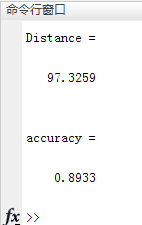5. 类簇中心点的选取
KMeans算法本身思想比较简单，但是合理的确定K值和K个初始类簇中心点对于聚类效果的好坏有很大的影响。最简单的确定初始类簇中心点的方法是随机产生数据大小范围内的K个点作为初始的簇类中心点。随机产生初始点并进行测试的程序代码如下
clear
data=load('Iris.txt');
data=data(:,2:end);
K=3;

%% 产生随机初始点
[numOfData,numOfAttr]=size(data);   % numOfData是数据个数，numOfAttr是数据维数

centroids=zeros(K,numOfAttr);       % 随机初始化，最终迭代到每一类的中心位置
maxAttr=zeros(numOfAttr);        % 每一维最大的数
minAttr=zeros(numOfAttr);        % 每一维最小的数
for i=1:numOfAttr
maxAttr(i)=max(data(:,i));    % 每一维最大的数
minAttr(i)=min(data(:,i));    % 每一维最小的数
for j=1:K
centroids(j,i)=maxAttr(i)+(minAttr(i)-maxAttr(i))*rand();  % 随机初始化，选取每一维[min max]中初始化
end
end

[Idx,C,distance]=KMeans(data,K,centroids,500);% 调用KMeans
Distance=sum(distance)% 计算类内距离之和

%% 计算准确率
c1=Idx(1:50,1);c2=Idx(51:100,1);c3=Idx(101:150,1);
Accuracy=(sum(c1==mode(Idx(1:50,1)))+sum(c2==mode(Idx(51:100,1)))+sum(c3==mode(Idx(101:150,1))))/numOfData

可以多运行几次以上代码，可以看出由于初始点事随机选取的每次运行得到的结果有所差异。这也是基本Kmeans算法的一个缺点，随着众多改进算法的提出Kmeans算法的这一问题也得到改善，深入了解的朋友可以查阅相关论文。
6. 结束语
本博文的完整MATLAB程序文件与整理好的数据集文件已经上传，下载即可运行。下载地址如下
点击下载：本文Kmeans算法M函数及测试完整文件
【公众号获取】
本人微信公众号已创建，扫描以下二维码并关注公众号“AI技术研究与分享”，后台回复“KM20180516”即可获取全部资源文件信息。由于编者能力有限，代码即使经过了多次校对，也难免会有疏漏之处。希望您能热心指出其中的错误，以便下次修改时能以一个更完美更严谨的样子，呈现在大家面前。同时如果有更好的实现方法也请您不吝赐教。


展开全文人工智能
• “如果把人工智能比作一块大蛋糕，监督学习只是上面的一层奶油“。日常生活中，从人脸识别、语音识别到搜索引擎，我们...聚类算法KMeans是无监督学习的杰出代表之一。本文是记录自己过去学习KMeans算法的系统小结，...“如果把人工智能比作一块大蛋糕，监督学习只是上面的一层奶油“。日常生活中，从人脸识别、语音识别到搜索引擎，我们看到越来越多人工智能领域的算法逐渐走向落地。尽管全球每日新增数据量以PB或EB级别增长，但是大部分数据属于无标注甚至非结构化。所以相对于监督学习，不需要标注的无监督学习蕴含了巨大的潜力与价值。聚类算法KMeans是无监督学习的杰出代表之一。本文是记录自己过去学习KMeans算法的系统小结，将从“KMeans简介，优缺点与优化策略，结合EM算法解释KMeans以及手推KMeans”几个方面来尽可能彻底、清晰地搞明白这个算法，希望对大家能有所帮助。一、聚类与KMeans与分类、序列标注等任务不同，聚类是在事先并不知道任何样本标签的情况下，通过数据之间的内在关系把样本划分为若干类别，使得同类别样本之间的相似度高，不同类别之间的样本相似度低（即增大类内聚，减少类间距）。聚类属于非监督学习，K均值聚类是最基础常用的聚类算法。它的基本思想是，通过迭代寻找K个簇（Cluster）的一种划分方案，使得聚类结果对应的损失函数最小。其中，损失函数可以定义为各个样本距离所属簇中心点的误差平方和：其中代表第个样本，是所属的簇，代表簇对应的中心点，是样本总数。二、具体步骤KMeans的核心目标是将给定的数据集划分成K个簇（K是超参），并给出每个样本数据对应的中心点。具体步骤非常简单，可以分为4步：（1）数据预处理。主要是标准化、异常点过滤。（2）随机选取K个中心，记为（3）定义损失函数：（4）令t=0,1,2,... 为迭代步数，重复如下过程知道收敛：           （4.1）对于每一个样本，将其分配到距离最近的中心（4.2）对于每一个类中心k，重新计算该类的中心KMeans最核心的部分就是先固定中心点，调整每个样本所属的类别来减少；再固定每个样本的类别，调整中心点继续减小。两个过程交替循环，单调递减直到最（极）小值，中心点和样本划分的类别同时收敛。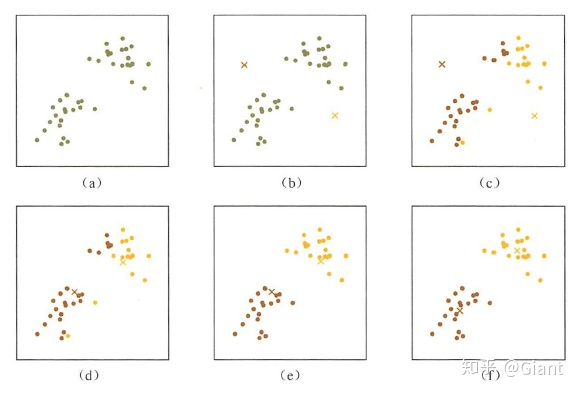KMeans迭代示意图三、优缺点与优化方法KMenas的优点： 高效可伸缩，计算复杂度 为接近于线性（N是数据量，K是聚类总数，t是迭代轮数）。收敛速度快，原理相对通俗易懂，可解释性强。KMeans也有一些明显的缺点：受初始值和异常点影响，聚类结果可能不是全局最优而是局部最优。K是超参数，一般需要按经验选择样本点只能划分到单一的类中根据以上特点，我们可以从下面几个角度对算法做调优。数据预处理：归一化和异常点过滤KMeans本质上是一种基于欧式距离度量的数据划分方法，均值和方差大的维度将对数据的聚类结果产生决定性影响。所以在聚类前对数据（具体的说是每一个维度的特征）做归一化和单位统一至关重要。此外，异常值会对均值计算产生较大影响，导致中心偏移，这些噪声点最好能提前过滤。2.合理选择K值K值的选择一般基于实验和多次实验结果。例如采用手肘法，尝试不同K值并将对应的损失函数画成折线。手肘法认为图上的拐点就是K的最佳值（上图对应K=3）。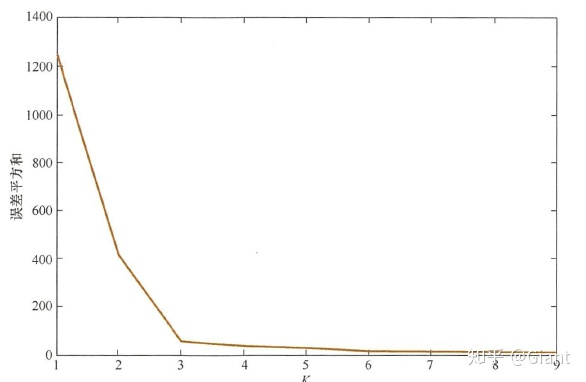手肘法为了将找寻最佳K值的过程自动化，研究人员提出了Gap Statistic方法。它的有点是我们不再需要肉眼判断，只需要找到最大的Gap Statistic对应的K即可。沿用第一节中损失函数记为，当分为K类时，Gap Statistic定义为：。是的期望，一般由蒙特卡洛模拟产生。我们在样本所在的区域内按照均匀分布随机地产生和原始样本数一样多的随机样本，并对这个随机样本做KMeans，得到一个，重复多次就可以计算出的近似值。的物理含义是随机样本的损失与实际样本的损失之差。Gap越大说明聚类的效果越好。一种极端情况是，随着K的变化几乎维持一条直线保持不变。说明这些样本间没有明显的类别关系，数据分布几乎和均匀分布一致，近似随机。此时做聚类没有意义。3.改进初始值的选择之前我们采取随机选择K个中心的做法，可能导致不同的中心点距离很近，就需要更多的迭代次数才能收敛。如果在选择初始中心点时能让不同的中心尽可能远离，效果往往更好。这类算法中，以K-Means++算法最具影响力。4.采用核函数主要思想是通过一个非线性映射，将输入空间中的数据点映射到高位的特征空间中，并在新的空间进行聚类。非线性映射增加了数据点线性可分的概率（与SVM中使用核函数思想类似）对于非凸的数据分布可以达到更为准确的聚类结果。四、从EM算法解释KMeansEM（Expectation-Maximum）算法即期望最大化算法，是最常见的隐变量估计方法。EM算法是一种迭代优化策略，每一次迭代都分为两步：期望步（E）、极大步（M）。EM算法的提出最初是为了解决数据缺失情况下的参数估计问题，基本思想是首先根据已有的观测数据，通过极大似然估计估计出模型的参数；再根据上一步估计出的参数值估计缺失数据的值；最后根据估计出的缺失数据和原有的观测数据重新对参数值进行估计，反复迭代直到收敛。EM算法基础和收敛有效性等问题可以参考Dempster、Laird和Rubin三人于1977年所做的文章《Maximum likelihood from incomplete data via the EM algorithm》。KMeans算法等价于用EM算法求解以下含隐变量的最大似然问题：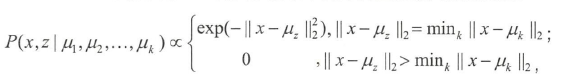其中是模型的隐变量，可以理解为当样本离第个类的中心点距离最近是，概率正比于，否则为0。在E步骤，计算：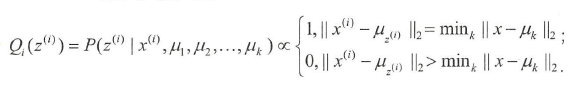等同于在KMeans中对于每一个点找到离当前最近的类。在M步骤，找到最优的参数，使得似然函数最大（假设对应的分布为，且满足）：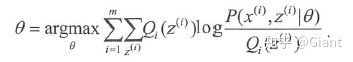经推导得：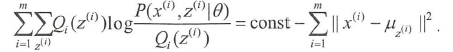这一步等价于找到最优的中心点，使得损失函数达到最小。此时每个样本对应的类已确定，每个类对应的最优中心点可以由该类所有点取平均值得到。这与KMeans算法中根据当前类的分配更新聚类中心的步骤等同。五、手推KMeans最后，为大家提供一个pyTorch手推实现KMeans的代码（通过sklearn包也能方便调用），结合理论梳理一遍具体实现，相信可以理解的更为扎实。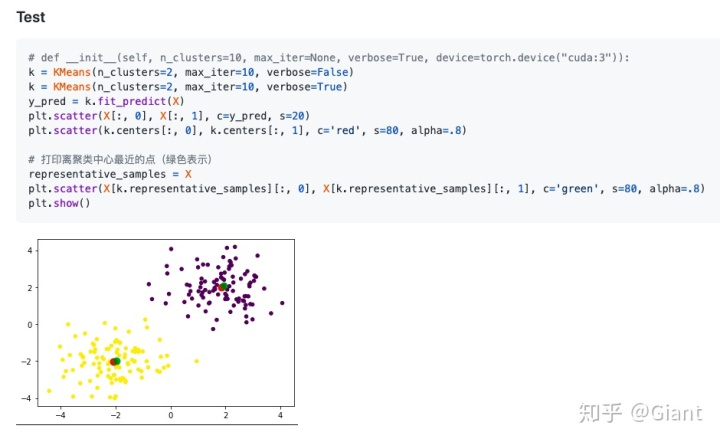小结KMeans作为一种无监督聚类算法，在日常生活中有大量应用。经过适当的预处理，可以对数据做初步分析，甚至挖掘出隐含的价值信息（例如对用户日志做聚类，得到一些高频高质量的新FAQ）。相比于SVM、GBDT等机器学习算法，理解起来相对通俗易懂，实乃实在又实用。Reference：1.《百面机器学习》5.1 K均值聚类：P92-P1012.EM算法详解3.手推KMeans
展开全文• kmeans算法就是要将这个集合分成k类，每一类有一个中心，其中k的大小是人为设置的。 kmeans工作流程如下： 1. 随意选k个点作为每个类初始的中心点 2. 遍历每一个点i，分别计算点i到这k个点的距离，离哪一个点...
原理 ：##
一个含n个元素的集合D：{x1, x2, …, xn}，其中xi = (xi1,xi2,…,xir)，即表示每个元素有r个属性（纬度）。kmeans算法就是要将这个集合分成k类，每一类有一个中心，其中k的大小是人为设置的。
kmeans工作流程如下：
随意选k个点作为每个类初始的中心点
遍历每一个点i，分别计算点i到这k个点的距离，离哪一个点最近就将点i划分成哪一类
所有的点都划分完后，求每一类所有点的平均值，作为该类新的中心点
然后重复2，3直到中心点不在变化，或者重复2，3到一定次数
优缺点：
优点：简单，高效
缺点：需要人为设置聚类的个数；对一些不规则的分布聚类效果很差；对异常值十分敏感，如下图：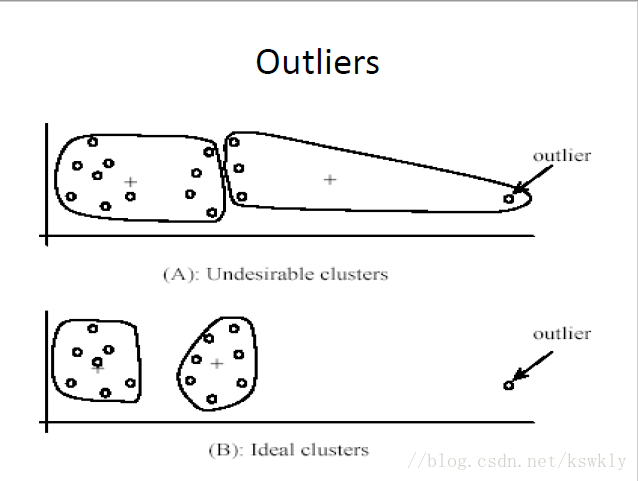MATLAB实现
源数据链接：https://pan.baidu.com/s/1aioiSIkvdN53AU1lzAScrw
提取码：df1g，若失效，可从资源链接中直接获取
代码：
[b] = xlsread('aggregate.xlsx',1,'A1：c788');
x = b(:,1);
y = b(:,2);
%c = b(:,3);

data = [x(1:7,1),y(1:7,1)];
%用于记录点到样本的距离
dist = zeros(1,7);
for k = 1:300
%用来记录点被分到那个类中
c = zeros(788,1);
sum = zeros(7,3);
for i = 1:788
for j = 1:7
dist(1,j) = sqrt((x(i,1)-data(j,1))^2+(y(i,1)-data(j,2))^2);
end
[mi,index]=min(dist);
c(i,1) = index;
sum(c(i,1),1)=x(i)+sum(c(i,1),1);
sum(c(i,1),2)=y(i)+sum(c(i,1),2);
sum(c(i,1),3)=sum(c(i,1),3)+1;
end
%重新计算均值
for m = 1:7
data(m,1) = sum(m,1)/sum(m,3);
data(m,2) = sum(m,2)/sum(m,3);
end
end
%画图
for i = 1:788
rand('seed',c(i,1));
color = rand(1,3);
plot(x(i,1),y(i,1),'*','color',color);
hold on;
end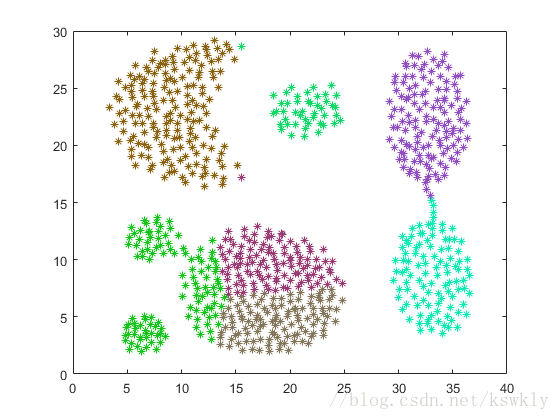展开全文• kmeans聚类算法是一种简单实用的聚类算法，matlab自带函数kmeans可直接对数据进行kmeans聚类。为了方便更好地掌握kmeans聚类算法，今天我们自己来实现一个弱化的版本mykmeans。mykmeans输入包含三项，分别为聚类所...
• kmeans聚类算法是一种简单实用的聚类算法，matlab自带函数kmeans可直接对数据进行kmeans聚类。为了方便更好地掌握kmeans聚类算法，今天我们自己来实现一个弱化的版本mykmeans。 mykmeans输入包含三项，分别为聚类所...
• k-means算法是非监督聚类最常用的一种方法，因其算法简单和很好的适用于大样本数据，广泛应用于不同领域，本文详细总结了k-means聚类算法原理 。目录1. k-means聚类算法原理2. k-means聚类算法步骤3. k-means++聚类...
• ## kmeans聚类算法及matlab实现

万次阅读 多人点赞 2015-11-12 19:25:10
kmeans聚类算法介绍：kmeans算法是一种经典的无监督机器学习算法，名列数据挖掘十大算法之一。作为一个非常好用的聚类算法，kmeans的思想和实现都比较简单。kmeans的主要思想：把数据划分到各个区域(簇)，使得数据与...数据挖掘
• Kmeans聚类算法详解与实现，Kmeans算法的MATLAB实现、python实现源代码都有。附有算法原理的解析。 对应的博客地址：http://blog.csdn.net/zengxiantao1994/article/details/73441922
• kmeans聚类算法是一种简单实用的聚类算法，matlab自带函数kmeans可直接对数据进行kmeans聚类。为了方便更好地掌握kmeans聚类算法，今天我们自己来实现一个弱化的版本mykmeans。mykmeans输入包含三项，分别为聚类所...kmeans算法 kmeans聚类算法
• ## Kmeans聚类算法及其matlab源码

万次阅读 多人点赞 2016-10-24 14:57:34
本文介绍了K-means聚类算法，并注释了部分matlab实现的源码。
• 使用MATLAB语言实现Kmeans聚类算法，并用并行工具箱进行加速运算。 function [cluster,label] = calculateDistforGpu(data,centeroids,max_iter) [m,n]=size(data); fprintf('m=%d,n=%d\n',m,n); % 获得类别个数 k ...图像处理
• Kmeans聚类算法matlab源代码，可用于图像分割等数字图像处理领域。
• 本文介绍了K-means聚类算法，并注释了部分matlab实现的源码。 </p><h2><a name="t0"></a>K-means算法</h2><p><span></span>K-means算法是一种硬聚类算法，根据数据到聚类中心的某种距离来作为判别该数据所属类别...
• kmeans(K均值聚类)是一种常用的聚类算法，因其简单且性能还算良好而受广泛应用。 kmeans聚类的中心思想是：确定k个类，计算模式特征矢量到每个聚类中心的“距离”，将特征矢量归并到距离最小的聚类中心所在的类中。...
• （2）掌握kmeans聚类算法的原理与实现。 实验内容 1、数据见 data.mat，编程实现 K means 算法代码 K_MeansMt，并写出详细注释。 测试代码如下： load 'data.mat'; [u re]=K_MeansMt(data,3); %最后产生簇标号 re ...python java 机器学习
• 这是我练习的第一个机器学习的算法，写的比较简单，肯定也有一些小错误。也参看了很多其他人的代码。现在贴出来算是我学习的一个历程啦。 clear all;close all;clc; data1=normrnd(0,0.25,100,2); %生成符合 data2=...
• kmean算法代码 function [Idx,C,D]=my_kmeans(X,c) %%k-均值分类 %输入:X样本集 c聚类簇数 %输出:Idx聚类标志 C聚类中心 D每个点到质心的聚类 [m,n]=size(X);%样本数m,样本维数 %从X中随机选择选择c个样本作为初始...
• K-means算法是非监督学习(unsupervised learning)中最简单也是最常用的一...本文章介绍K-means聚类算法的思想，同时给出在matlab环境中实现K-means算法的代码。代码使用向量化(vectorization1)来计算，可能不是很直...
• ## Kmeans聚类-matlab实现

千次阅读 2019-04-26 20:16:39
算法初始随机选取的三个样本，按照书上选的运行测试代码，可根据需求对代码进行改写，详细过程如下： 1、data.txt 数据集 0.697 0.460 0.774,0.376 0.634,0.264 0.608,0.318 0.556,0.215 0.403,0.237 0.481,0.149 0....
• 主要介绍Kmeans聚类算法的数学原理，并使用matlab编程实现Kmeans的简单应用。
• 在上一篇文章中，笔者介绍了聚类算法的主要思想与原理，并且还得到了其对应的目标函数。在接下来的这篇文章中笔者就开始介绍聚类算法的求解过程，以及其对应的代码实现。1 目标函数求解由上一篇文章的内容可知，聚类...kmeans算法 kmeans聚类算法
• 1、问题导入假如有这样一种情况，在一天你想去某个城市旅游，这个城市里你想去的有70个地方，现在你只有每一个地方的地址，这个地址列表很长，有70个位置。事先肯定要做好攻略，...而本文所提供的k-means聚类分析方...
• 所谓聚类分析，就是给定一个元素集合D，其中每个元素具有n个观测属性，对这些属性使用某种算法将D划分成K个子集，要求每个子集内部的元素之间相似度尽可能高...聚类算法有很多种，如K-means(K均值聚类)、K中心聚类、...聚类分析数据集
• 这是一种超快速MATLAB实现的kmeans聚类算法。This is a super duper fast implementation of the kmeans clustering algorithm.代码是完全向量化的，非常简洁。The code is fully vectorized and extremely succinct...
• 题记：最近有幸参与了一个机器学习的项目，我的主要工作是帮助进行数据预处理，期间用Python实现了K-means聚类算法，感觉收获很多特此记录下来和大伙儿分享。一机器学习项目的主要流程　机器学习项目的主要流程有...kmeans算法 kmeans聚类算法
• K-means聚类算法采用的是将N*P的矩阵X划分为K个类，使得类内对象之间的距离最大,而类之间的距离最小。 使用方法： Idx=Kmeans(X,K) [Idx,C]=Kmeans(X,K)  [Idx,C,sumD]=Kmeans(X,K)  [Idx,C,sumD,D]=Kmeans...
• 这是一种超快速MATLAB实现的kmeans聚类算法。 This is a super duper fast implementation of the kmeans clustering algorithm. 代码是完全向量化的，非常简洁。 The code is fully vectorized and extremely ...
• kmeans图像分割 该程序获取图像和所需的分区数，并找到不同类的平均值，并提供分类图像获取源代码...

# kmeans聚类算法matlabmatlab 订阅